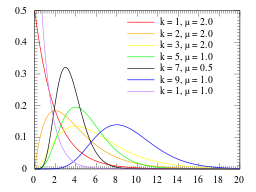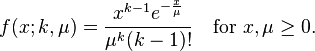# Erlang Distribution: Definition, Examples

Share on

Probability Distributions > Erlang Distribution

## What is the Erlang Distribution?

The Erlang distribution (sometimes called the Erlang-k distribution) was developed by A.K. Erlang to find the number of phone calls which can be made simultaneously to switching station operators. Erlang was a telecommunications engineer for the Copehagen Telephone Company; his formulas for loss and waiting time were used by many telephone companies, including the British Post Office. Erlang’s distribution has since been expanded for use in queuing theory, the mathematical study of waiting in lines. It is also used in stochastic processes and in mathematical biology.

The Erlang distribution is a specific case of the Gamma distribution. It is defined by two parameters, k and &u;, where:

• k is the shape parameter. This must be a positive integer (an integer is a whole number without a fractional part). In the Gamma distribution, k can be any real number, including fractions.
• μ is the scale parameter. Must be a positive real number (a real number is any number found on the number line, including fractions).Erlang distribution showing various possible shape and scale parameters. Image: IkamusumeFan|Wikimedia Commons.

The probability distribution function of the Erlang distribution is:The factorial(!) in the denominator is the reason why the distribution is only defined for positive numbers. An equivalent form of the pdf for this distribution includes λ, a measure of rate, which is related to μ in the following way:
μ = 1/λ.
λ represents the number of items or calls expected in a given amount of time.

## Relationship to Other Distributions

CITE THIS AS:
Stephanie Glen. "Erlang Distribution: Definition, Examples" From StatisticsHowTo.com: Elementary Statistics for the rest of us! https://www.statisticshowto.com/erlang-distribution/
---------------------------------------------------------------------------Need help with a homework or test question? With Chegg Study, you can get step-by-step solutions to your questions from an expert in the field. Your first 30 minutes with a Chegg tutor is free!